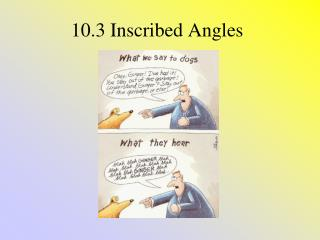Download Presentation10.3 Inscribed Angles

# 10.3 Inscribed Angles - PowerPoint PPT PresentationDownload Presentation## 10.3 Inscribed Angles

- - - - - - - - - - - - - - - - - - - - - - - - - - - E N D - - - - - - - - - - - - - - - - - - - - - - - - - - -
##### Presentation Transcript

1. 10.3 Inscribed Angles

2. Definitions • Inscribed Angle – An angle whose vertex is on a circle and whose sides contain chords of the circle • Intercepted Arc – the arc that lies in the • interior of an inscribed angle and has • endpoints on the angle (arc AB)

3. Theorems • If an angle is inscribed in a circle, then its measure is half the measure of the intercepted arc. • m < APB = ½ AB • m < APB = 60

4. Theorems • If two inscribed angles of a circle intercept the same arc, then the angles are congruent. • m<DCR = m<RAD

5. Theorems 3. If a right triangle is inscribed in a circle, then the hypotenuse is a diameter of the circle.

6. Last Theorem!!!! 4. A quadrilateral can be inscribed in a circle if and only if its opposite sides are supplementary.

7. Examples • Find the measure of arc TR • 260 • Find the m<DIS • 21

8. Last Example 3. Find the measure of angle D and angle W

9. Homework • P. 617 9-23all